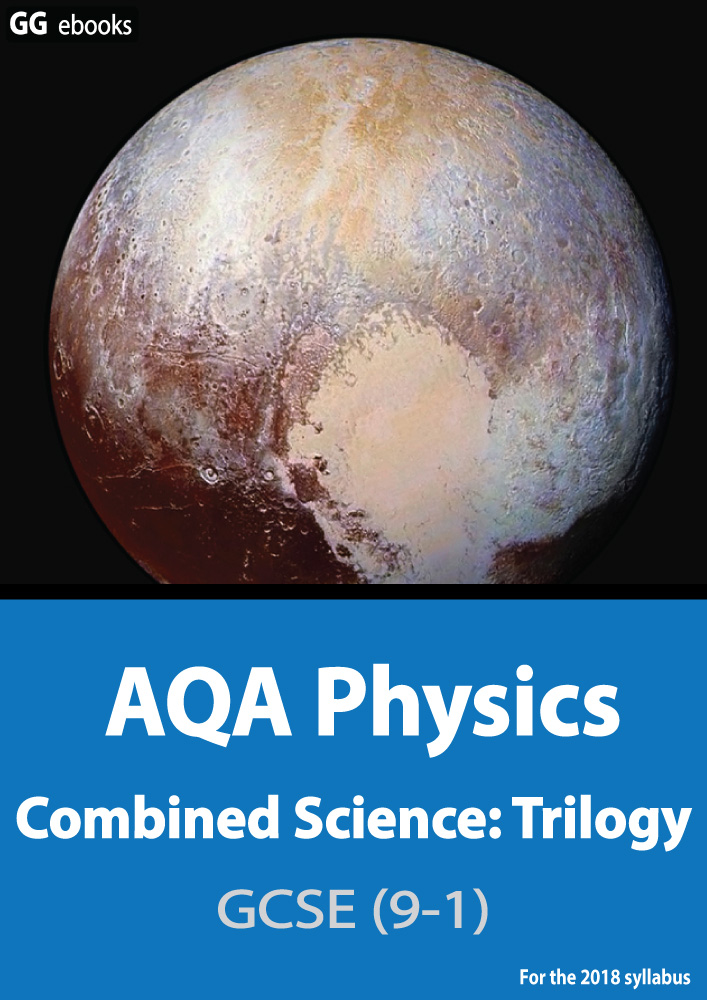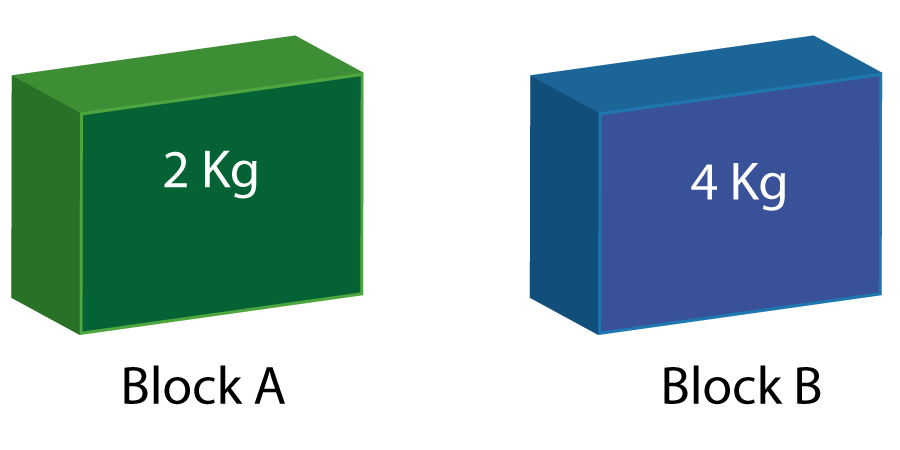NEED HELP? AQA GCSE digital textbooks From the makers of Grade Gorilla GGebooks.com(Opens in a new tab)1. Which of these sentences is true?
• A). In a gas the particles have strong forces between them.
• B). Solids take the shape of the container they are in.
• C). The forces are strong between particles in a solid.
• D). Particles in a liquid move faster than in a gas.Which of these descriptions matches which state(s) of matter?

2. Particles are widely separated.

3. No fixed shape.

4. Strong bonds hold the particles firmly in place.

5. Which answer best describes the difference between these 2 blocks?• A) Block A has a larger volume than block B
• B) Block B is denser than block A
• C) Block A has more mass than block B
• D) Block A has more tightly packed particles than block B

6. What is the formula for density?

• A) Density = volume x mass
• B) Density = volume / mass
• C) Density = mass / volume
• D) Density =  ½ x mass x (volume)

7. A liquid is added to a measuring cylinder to find the density. What is the correct calculation to find the mas of the liquid?

• A) (mass of cylinder) + (mass of liquid and cylinder)
• B) ( mass of cylinder and liquid) - (mass of water)
• C) (mass of cylinder) - (mass of liquid and cylinder)
• D) ( mass of cylinder and liquid) - (mass of cylinder)

8-13:

This table shows data for various blocks of different substances. What are the missing values?Mass (g) length (cm) width (cm) height (cm) Volume (cm3) Density (g/cm3) 8. 20 2.5 2 5 25 ? 500 50 0.8 1.25 9. ? 324 4 0.25 25 3 3 4 36 9 10.+11. 400 4 5 8 ? 100 160 800 1000 ? 0.4 2.5 25 40 12.+13. ? 10 100 0.1 36.1 361 3.8 ? 0.2 0.5 2 5 1 1.9 19